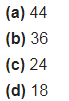# Express the following as the sum of two odd primes (a) 44

Express the following as the sum of two odd primes